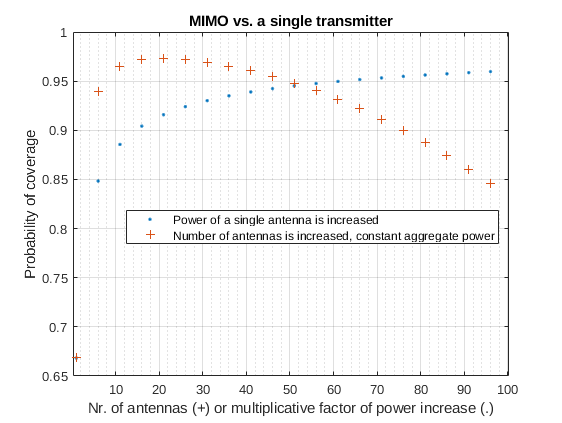## June – MIMO

Multiple-input and multiple-output (MIMO) antenna technology is used to exploit the multi-path propagation to improve the capacity of a communication link (i.e. more gigabytes of internet speed). In principle, capacity can always be increased merely by increasing the power of the transmitting antenna. However – apart from being energy consuming – this increases also interference to any other receivers should they operate in the same frequency band. In MIMO the energy is divided among multiple antennas, and the link capacity is improved without using any extra energy and without increasing the interference to other transmitters.

But how and why does it work? Here is how I came up with a simple argument based on stochastic geometry.

Let us make few assumptions. We assume a flat and infinite Earth (no tin-foils). In addition we assume that our communication channel environment consists of many obstacles so that our transmitting antenna and receiving antenna cant see each other. We can assume that we are in a city full of houses, cars, trees, etc.. Then our data signal is prone to propagate to the receiver through multiple paths; for example through different streets around different houses. Aggregate signal in the receiver will be Rayleigh faded. City is full of mobile phones transmitting data with their base-stations, and in the other hand causing interference to each other. We make a natural assumption that the interfering transmitters are distributed according to the Poisson point process.

As derived in here we express the probabilityof a successful transmission (for example a message to a friend) as:wheredenotes the mean transmitting power.

In MIMO we use multiple antennas to send the message. In each antenna the message should be coded in a way that it can't be mixed with the information that the other antennas are transmitting. This can be done by orthogonal modulation. Assuming that each message from each antenna will propagate to the receiver in an independent way, we can calculate by complementary probability that at least one message is received:whereis the number of antennas. Notice that we divided the transmitting powerby number of antennas, so we don't increase the aggregate power at all. In the other hand, should we have no MIMO technology at hand, we could try to improve to increase the link quality just by increasing the power. In the following figure we compare these two cases.It is evident that MIMO is a great solution for increasing the throughput of a wireless communication link. Using MIMO we can achieve better data-rates than by increasing the power of the transmitters.

1
François Baccelli, Bartlomiej Blaszczyszyn Stochastic Geometry and Wireless Networks, Volume 2 - Applications